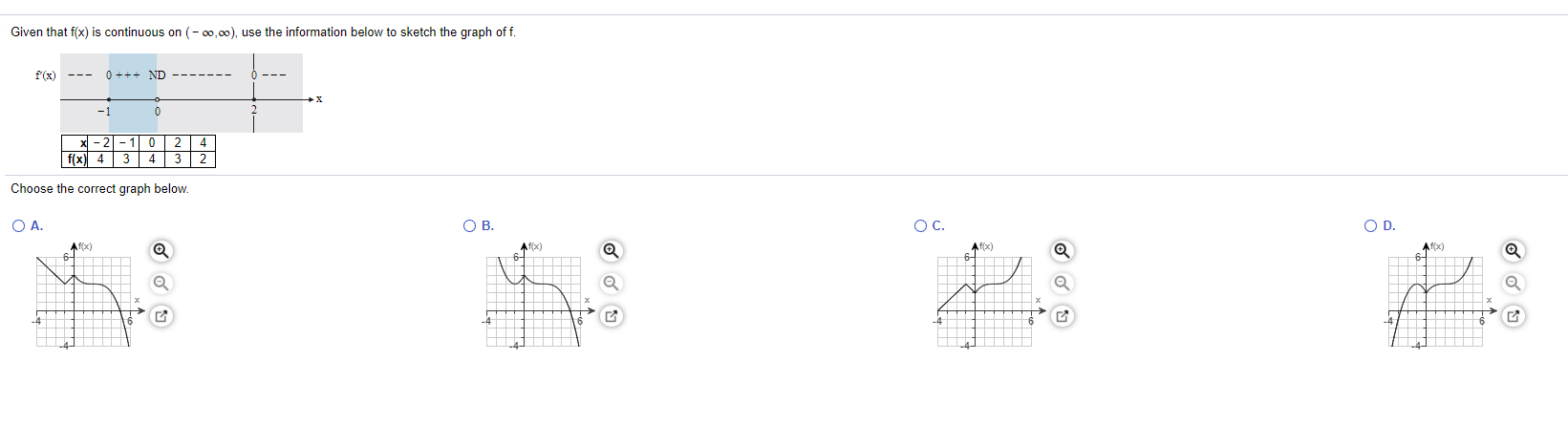### Create an Account

Already have account?

### Forgot Your Password ?

Home / Questions / Given that f(x) is continuous on (-00,00), use the information below to sketch the graph o...

# Given that f(x) is continuous on (-00,00), use the information below to sketch the graph off. 0 ++- ND 2 f(x) 4 3 2 Choose the correct graph below. OA. OB. O C. OD Af(x) 61 Af(x) 64 Af(x) Af(x)

Given that f(x) is continuous on (-00,00), use the information below to sketch the graph off. 0 ++- ND 2 f(x) 4 3 2 Choose the correct graph below. OA. OB. O C. OD Af(x) 61 Af(x) 64 Af(x) Af(x)Apr 14 2021 View more View Less

#### Answer (Solved)Subscribe To Get Solution